# The Atmospheric Lapse Rate and Molar Density

By Andy May, Michael Connolly and Ronan Connolly

In this post, we will discuss the tropopause, atmospheric molar density and the lapse rate (the change in atmospheric temperature with altitude). The key points are:

1. A change in the molar density versus pressure best-fit line is a change in the equation-of-state.
2. The NOAA and WMO definitions of the tropopause are different and somewhat clumsy.
3. Molar density plots are a better and more robust way to pick the tropopause.

The World Meteorological Organization (WMO) defines the tropopause as:

“The boundary between the troposphere and the stratosphere, where an abrupt change in lapse rate usually occurs. It is defined as the lowest level at which the lapse rate decreases to 2°C/km or less, provided that the average lapse rate between this level and all higher levels within 2 km does not exceed 2°C/km. (International meteorological vocabulary, as seen in Wikipedia)

The lapse rate is simply the change in temperature with height, it is often presented as positive when temperature decreases with height (as in the WMO quote above), but here we will present it as a negative number when temperature decreases with height. In the “standard atmosphere” it is assumed to be -6.5°C per kilometer below the tropopause. NOAA defines the tropopause as an abrupt change in the lapse rate to neutral (zero) or positive (temperature increasing with height). These definitions often don’t work well, so here we will discuss a new method of locating the tropopause using atmospheric molar density.

We have analyzed the IGRA weather balloon dataset of over 20 million balloons for lapse rate and other values. The dataset includes balloon flight records from the 1940’s to the present day. The stations in the dataset at four selected times are shown in figure 1.Figure 1

Global coverage is sparse in the 1940’s, but it fills in by 1960. The data collected includes atmospheric pressure, temperature, and relative humidity. Data is collected up to the point where the balloon bursts, generally an altitude of 30 to 40 km. In figures 2 and 3 we present the Northern Hemisphere summer (June-July-August) and winter (December-January-February) lapse rates computed from the balloon data in 15° latitude bands around the Earth.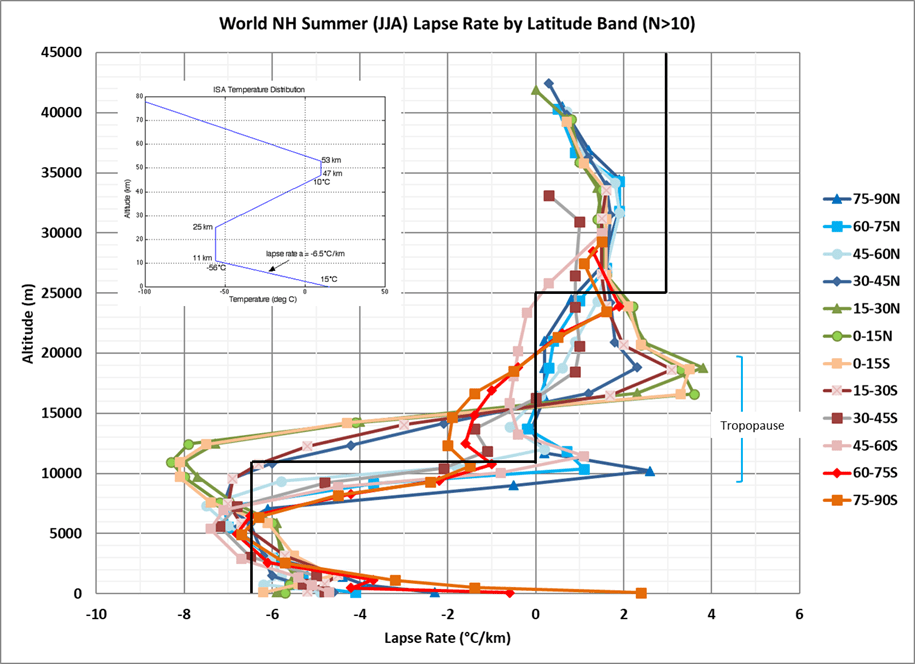Figure 2, Summer Lapse Rates, for each latitude band the tropopause is the sudden reversal of the lapse rate in the noted region.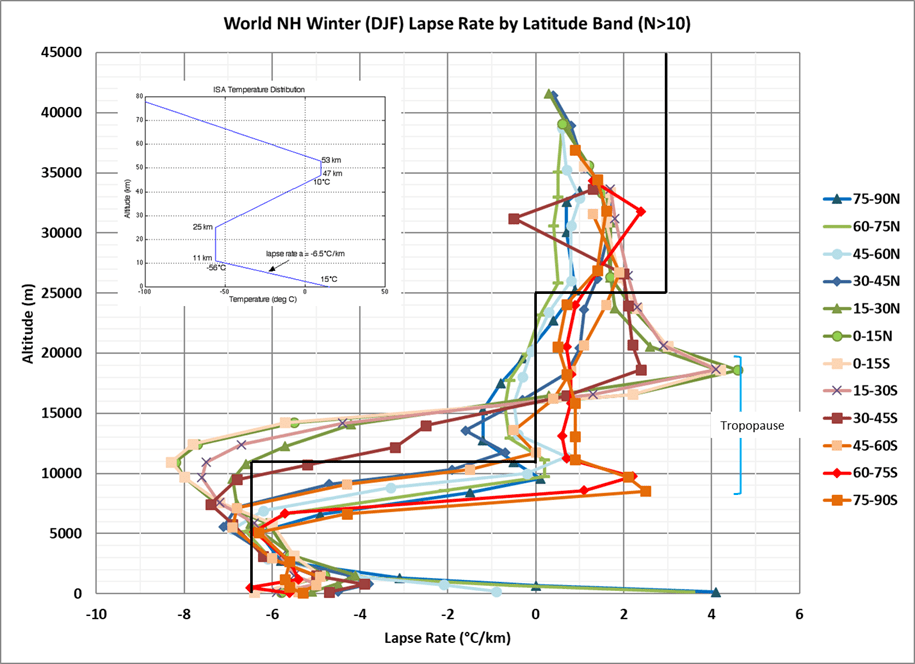Figure 3, Winter Lapse Rates, for each latitude band the tropopause is the sudden reversal of the lapse rate in the noted region.

In both figures the Southern Hemisphere rates are shown in oranges and reds. The Northern Hemisphere rates are shown in blues and greens. The inset figure is the international standard atmosphere (ISA) temperature distribution. The ISA temperature distribution is also plotted in the main graph as a heavy black line, so it can be compared to the data. As we can see the ISA lapse rate is only a very rough estimate of the average measured lapse rate. If we used the tropopause definition preferred by NOAA (a zero-lapse rate crossing) we can see that the various latitude bands cross this threshold between 8 and 18 km. After the lapse rate crosses zero, it typically reaches a local maximum and reverses direction. After it reverses direction it sometimes stays positive at about the same positive value. Other times, after the reversal, it returns to negative and then crosses the zero point again. Surface temperature inversions sometimes result in positive values at the surface.

Most of the points on the graphs are averages of over 10,000 balloon records, and some points are averages of well over 100,000 records. However, at very high altitudes (> 35 km) there are few records, so we don’t include any points on either graph with fewer than 10 records.

The two most southern winter (Northern Hemisphere summer) latitude bands (south of 60°S) do not cross the zero-lapse rate line until well into the stratosphere, thus using the NOAA definition they do not have a tropopause. However, both have a noticeable lapse rate reversal (see figure 2) at about 10 km and this point was used as the base of the tropopause since it meets the WMO tropopause definition (> -2°C/km).

The altitude displayed in figures 2 and 3 is computed from temperature and pressure readings. It is usually accurate to within 600 meters or so, but above 11 km it loses accuracy quickly and is potentially off by more than a kilometer. Some possible reasons for this are discussed in Connolly and Connolly 2014, paper 1 (section 4.1) and here. The tropopause occurs between about 8 km and 18 km depending upon the latitude and the surface temperature. It is higher in the tropics and mid-latitudes and lower in the polar regions. It is lowest in Antarctica in the Antarctic summer.

The height of the tropopause in the NH winter and summer is shown in figure 4. This is where the lapse rate first goes below zero, ignoring places where it is below zero at the surface, like in the polar regions during the winter. As mentioned above, the two points south of 60S in the NH summer are lapse rate reversals between -2 and zero (see figure 2).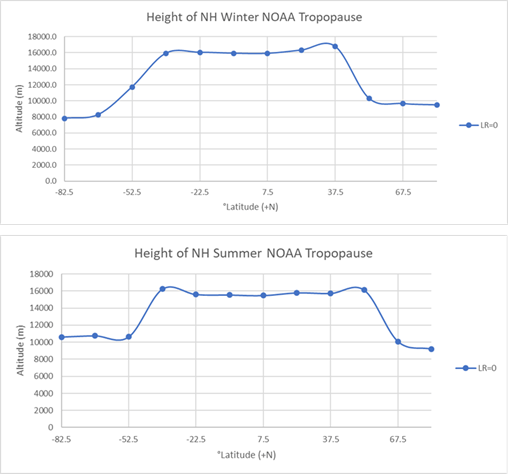Figure 4, height of the tropopause

Molar Density versus Lapse Rate

The weather balloons provide enough measurements to compute the molar density of the atmosphere, if we assume the ideal gas law. This is described in detail in Connolly and Connolly, 2014, paper 1, but we will summarize the key points here. Molar density is the number of moles of the atmosphere per cubic meter. Molar density or “D” is then n/V, where “n” is the number of moles and “V” is the volume. As Connolly and Connolly then show, D is also equal to P/(RT), where R=8.314 (the universal gas constant), “P” is pressure and “T” is temperature. So, all you need to do is divide the P (Pressure) values by the corresponding temperature multiplied by 8.314. One would expect a slope of 1/T, if we plot the molar density versus pressure as we have done for the Northern Hemisphere winter and summer in figure 5.Figure 5

In the top portion of each seasonal plot in figure 5 we have plotted the average molar density and lapse rate versus pressure for the latitude band from 30N to 45N. The lapse rate first crosses zero at 9,000 Pa and 11,000 Pa for winter and summer, respectively. The left-hand lower plots show the troposphere points, they have a slope of 0.0004 and a positive intercept greater than 3.3, with an R2 of 0.998 and 0.999, due to some minor curvature at the lower pressures (higher altitude). The remaining points are in the tropopause or stratosphere and they are plotted to the lower right for each season. The points have a slope very close 0.0006 and a very small intercept of -0.03 to -0.06. The tropopause here is roughly at 9,000 Pa or approximately 17 km in winter. In the summer it is roughly 11,000 Pa or 15.5 km. The troposphere and stratosphere lines are significantly different, suggesting a state-change in the gases. There is no change in gas composition at that altitude and temperature changes can’t account for the change in slope, since the slope is 1/T anyway.

The Connolly and Connolly, 2014, paper 2 suggests that the change taking place at the tropopause could be the formation of oxygen multimers. This is a possible explanation that fits the known data at this point. See the paper for more details.

The remarkable new information is that the slope changes at the tropopause. Figure 6 shows the same set of plots for the Southern Hemisphere latitude band from 30S to 45S in the Southern Hemisphere winter.Figure 6

In figure 6 we see that the line describing the troposphere is distinctly different from the line describing the tropopause and stratosphere. Both lines have an R2 of 0.999.

The Tropopause

The tropopause usually occurs at a temperature between 195K and 225K as seen in figure 7.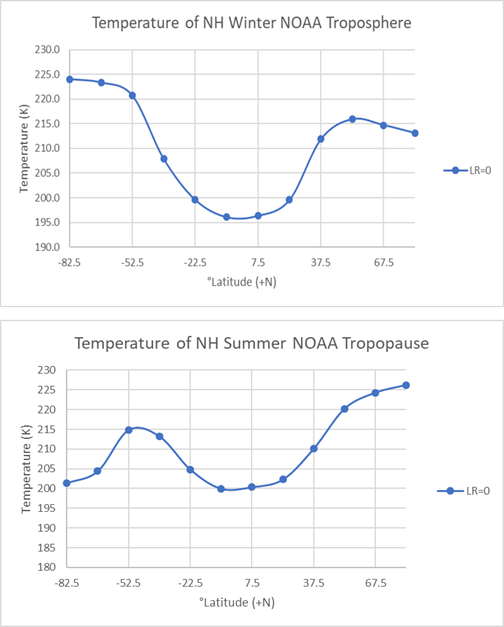Figure 7

The details of the conditions in the tropopause are discussed in Connolly and Connolly, 2014, paper 2. Plots of all the latitude bands are presented in their figure 4.

So far, we have used the conventional definitions of the tropopause. We’ve interrogated the atmospheric pressure, altitude and temperature profiles and interpreted a tropopause when we see a sudden reversal of the lapse rate from negative to “more positive.” The WMO chooses a -2°/km cutoff and NOAA chooses a zero-degree cutoff. In both cases, other reversals near the surface or in the stratosphere must be ignored. All these rules and exceptions make these definitions less than satisfying.

A simpler definition is possible using molar density. The molar density versus pressure line in the stratosphere intersects the molar density versus pressure line from the troposphere at the tropopause everywhere as far as we can tell. The lines fit the data so well that few points are required for an accurate result. Figure 8 is for the Antarctic region in winter. This is one of the previously mentioned latitude bands where the lapse rate does not cross zero as required by the NOAA definition. By using crossing molar density best fit lines we can establish a tropopause at the lapse rate reversal between -2 and zero; which is where we would put it using the WMO definition quoted at the beginning of the post. Solving the two best fit lines for their intersection we can show they cross at 22,576 Pa.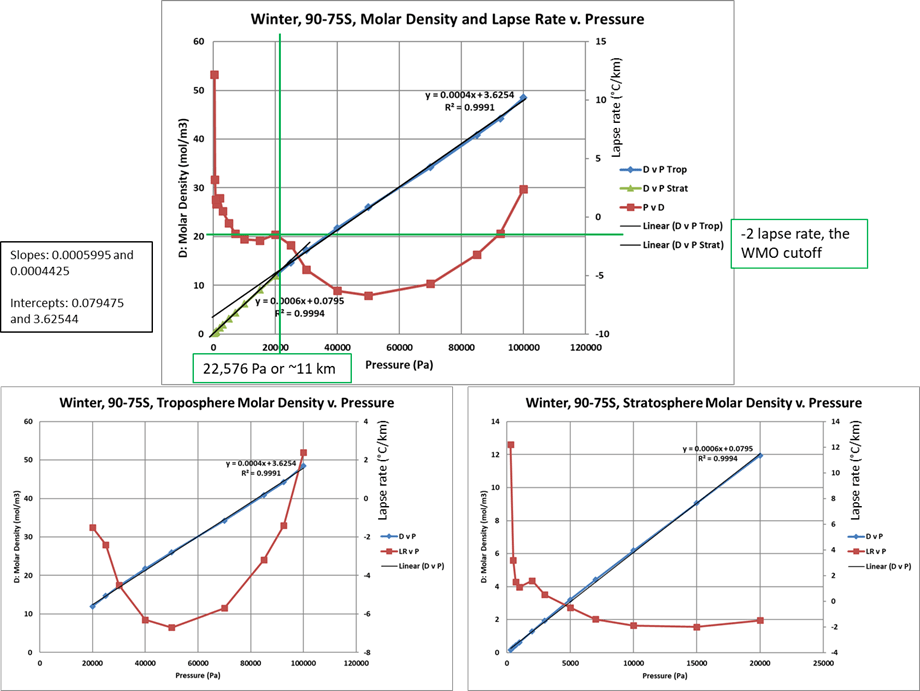Figure 8

Discussion

The data presented here shows that the tropospheric lapse rate is not the constant -6.5°C/km often assumed. The lapse rate curves vary considerably around the world and are never straight lines. It also appears that the atmospheric equation-of-state changes for some reason, possibly the formation of oxygen multimers, at the base of the tropopause.

While the NOAA tropopause definition works most of the time, the requirement for a lapse rate crossover at zero can be problematic. In some records, especially in the Antarctic in winter, the zero crossover is not reached; although the shape of the lapse rate versus altitude curve suggests a tropopause is present. In these circumstances the WMO definition works better. Both definitions are somewhat imprecise, and we suggest that molar density versus pressure best fit lines might locate the tropopause more precisely and rigorously. These lines can be created with points deeply in the stratosphere and deeply in the troposphere and the intersection of the lines would be the tropopause. There always seems to be a change of state at the tropopause that causes the slope and intercept of the lines to change abruptly. In some areas there is some apparent curvature near the boundary, but by choosing the points used to establish the lines above the tropopause and some distance below it, corruption by this transitional curvature can be avoided.

The tropopause is lower in the polar regions and higher between 40N and 40S latitude. This might be partly due to surface temperature, but the shape of the curves in figure 4 suggests that factors other than surface temperature are involved. As can be seen in figure 7 and in table 1 from Connolly and Connolly, paper 2, the temperature and pressure conditions at the tropopause vary considerably around the world.

Molar density is not often discussed in the meteorological literature to the best of our knowledge, but it greatly simplifies some atmospheric calculations. Temperature varies vertically in complex patterns, pressure trends can also be complex, but less so. Molar density is composed of two fundamental straight lines, one for the troposphere and one for the stratosphere and tropopause. There is a slight curvature between the two lines and more deeply in the troposphere there can be some slight curvature due to humidity changes or precipitation events. However, for any given location and time the detection of the slope and intercept for a molar density versus pressure plot is easy to detect and the fits are very good. This would seem to be a useful tool in meteorological and climate modeling.

While Andy May wrote this post, much of the technical work was done by Michael and Ronan Connolly.

Excel spreadsheets containing the data used to make the plots (and much more data) can be downloaded here.